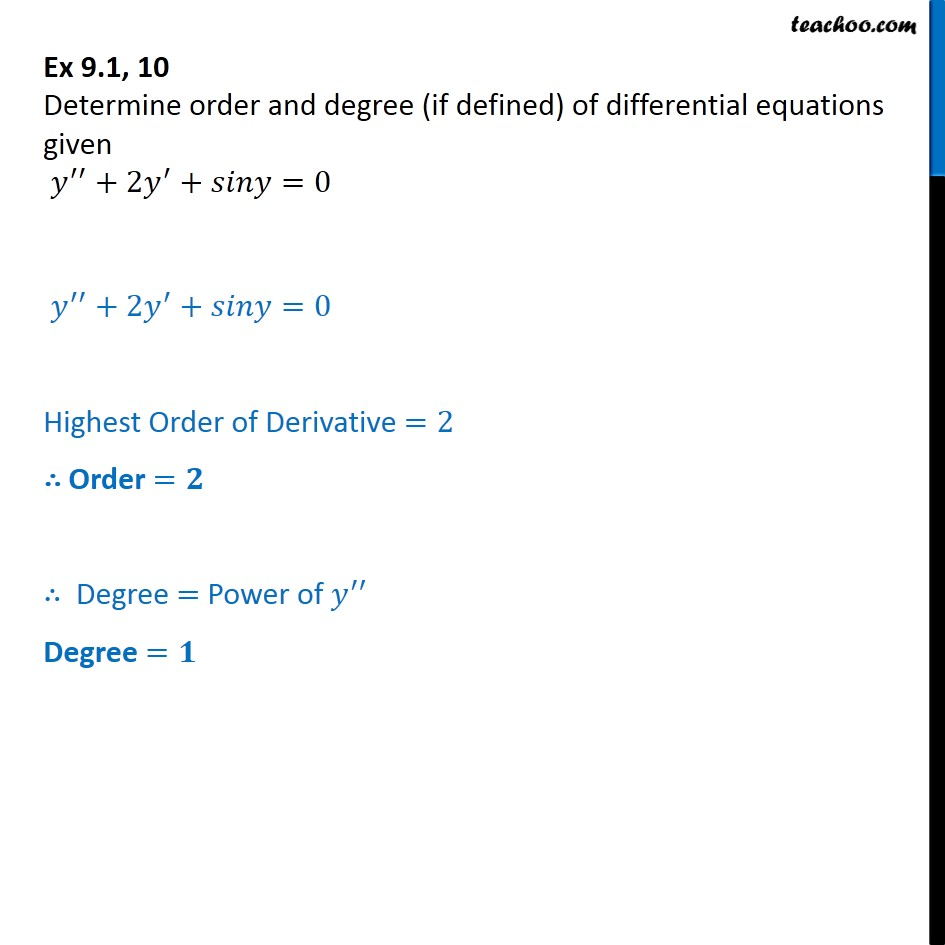1. Chapter 9 Class 12 Differential Equations
2. Serial order wise
3. Ex 9.1

Transcript

Ex 9.1, 10 Determine order and degree (if defined) of differential equations given y + 2y + = 0 y + 2y + = 0 Highest Order of Derivative =2 Order = Degree = Power of y Degree =

Ex 9.1